# 07-天气预报厂—《混沌之新数学》读书笔记

2020年5月6日 //

## 天气预报

1922年，理查森发表一篇报告《用数值方法进行天气预报》，构想如何用数学方法进行天气预报。其中有一个异想天开的幻想——天气预报厂——基于许多人操控计算器，最终实现实时的、甚至超前的天气预报。

1950年，美国 ENIAC 计算机就作出了天气预报方面首例成功的计算。1953年，普林斯顿 MANIAC 计算机表明，正常天气预报是完全可行的。

## 洛伦茨吸引子

1963年，洛伦茨发表了著名的论文《确定性非周期流》，在短短 12 页的论文中，洛伦茨预示了非线性动力学的若干主要思想，而这些都是在非线性动力学流行起来之前所做的。本文的摘要可简述如下：可以将流体动力学的解等同于相空间中的轨线，非周期解对小修正而言通常是不稳定的（书中翻译为稳定，属于翻译错误），以致略微不同的初始状态会演变为显著不同的状态；用数值方法进行求解时，发现所有的解都不稳定，几乎所有的解都是非周期解。

$$\frac{dx}{dt}=\sigma \left( y-x \right)$$ $$\frac{dy}{dt}=x\left( \rho -z \right) -y$$ $$\frac{dz}{dt}=xy-\beta z$$

• 若 $\rho<1$ 时，所有轨线都指向原点，即系统在原点处存在一个吸引子。由于没有任何其他吸引子，此吸引子也是全局吸引子。在原点处，系统没有对流。
• 若 $\rho=1$ 时，系统发生叉分岔，这种分岔是一个不动点变为三个不动点的一类局部分岔。
• 当 $\rho>1$ 时，出现了两个额外的吸引子，其位置分别是 $\left( \sqrt{\beta \left( \rho -1 \right)},\sqrt{\beta \left( \rho -1 \right)},\rho -1 \right)$ 和 $\left( -\sqrt{\beta \left( \rho -1 \right)},-\sqrt{\beta \left( \rho -1 \right)},\rho -1 \right)$。这两个额外的吸引子都对应于稳定对流。这两个平衡点仅仅在 $\rho <\sigma \frac{\sigma +\beta +3}{\sigma -\beta -1}$ 时才是稳定的。当 $\sigma$ = 10，$\beta$ = 8/3 时，$\rho$ 的临界值是 24.737。
• 若 $\rho =\sigma \frac{\sigma +\beta +3}{\sigma -\beta -1}$ 时，两个额外的吸引子发生分岔，其分岔类型是次临界霍普分岔。当 $\rho$ 大于此临界值后，这两个额外的吸引子消失（即失去稳定性）。
• 当 $\sigma$ = 10，$\beta$ = 8/3, $\rho$ = 28 时，系统表现出混沌特性（但不是所有初值的解都是混沌的）。几乎所有初始点都朝洛伦茨吸引子发展，

load(dynamics)$load(draw)$

/* System parameters */
a: 10; b: 8/3; r: 28;

lorenzSystem: [a*(y-x), -x*z+r*x-y, x*y-b*z];
dependentVariables: [x, y, z]$initialValues: [1, 1, 1]$
timeRange: [t, 0, 50, 0.01]$/* solution via 4th order Runge-Kutta method */ systemSolution: rk(lorenzSystem, dependentVariables, initialValues, timeRange)$
solutionPoints: map(lambda([x], rest(x)), systemSolution)$draw3d(point_type=none, points_joined=true, color=blue, xlabel="x(t)", ylabel="y(t)", zlabel="z(t)", points(solutionPoints));  以上代码中，位置初值为 (1, 1, 1)，改变其中的 r 变量（即$rho$）的值，可得到如下一系列轨线图。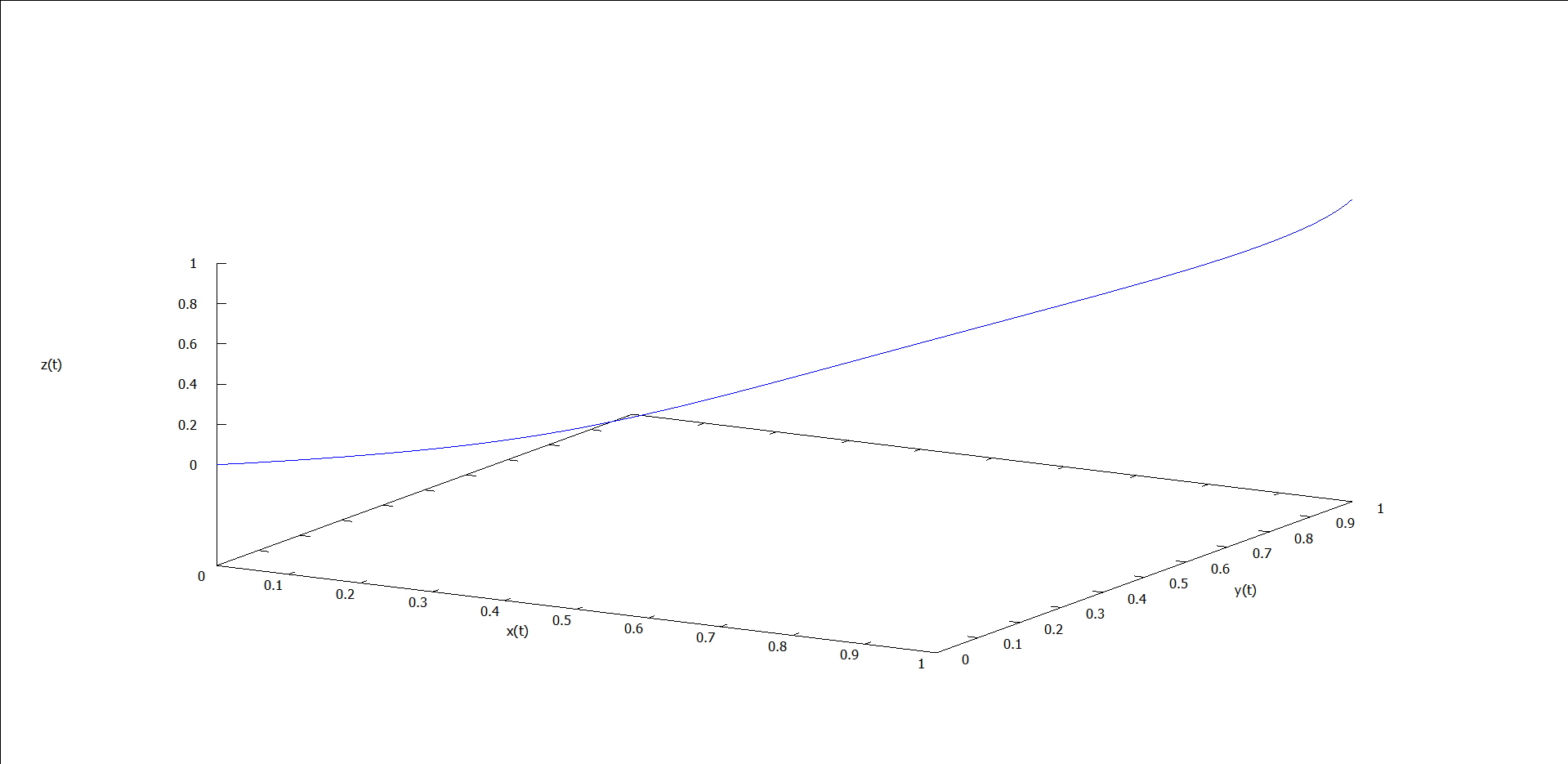图1 ρ = 0.5 时的运动轨线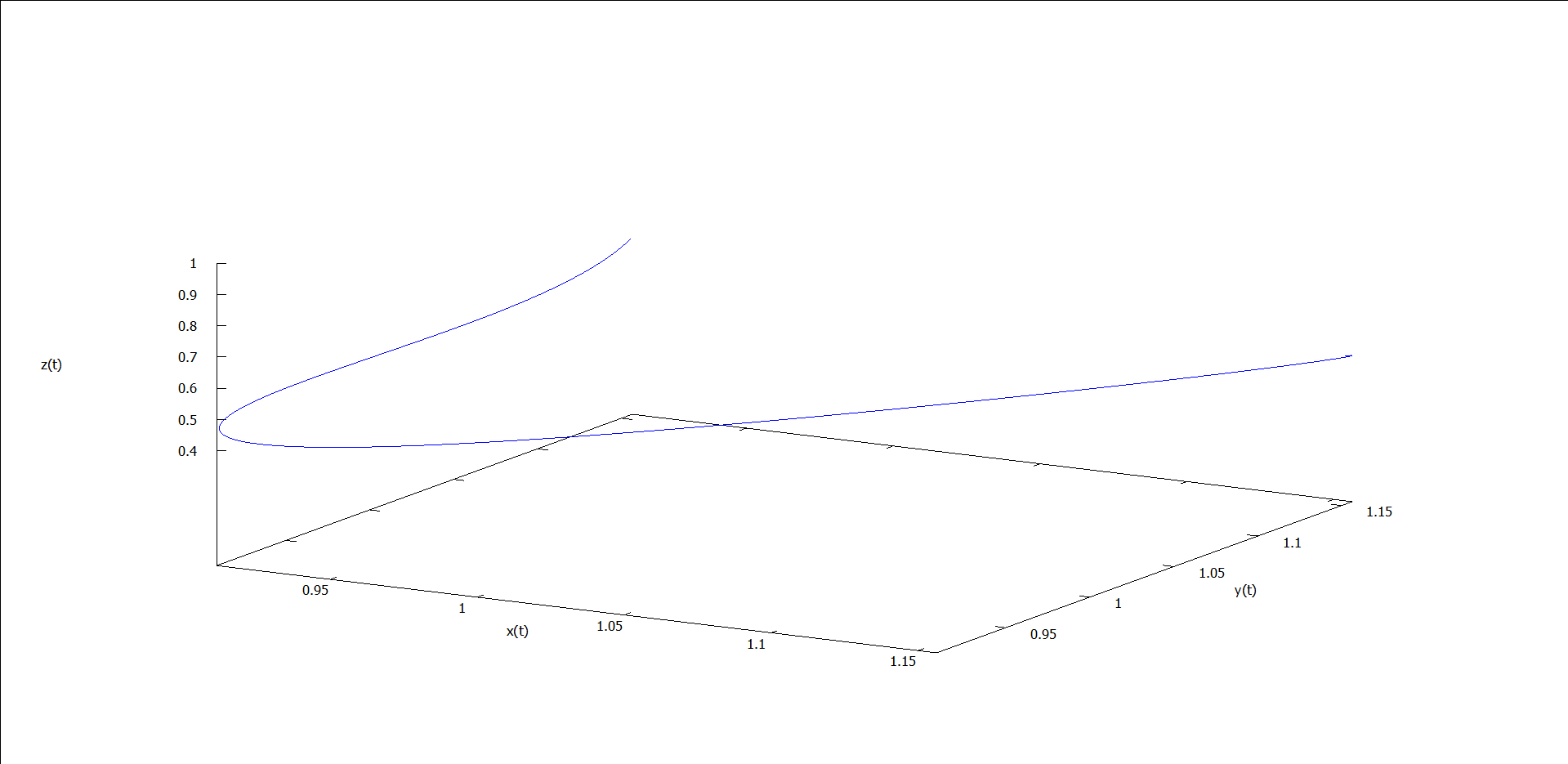图2 ρ = 1.5 时的运动轨线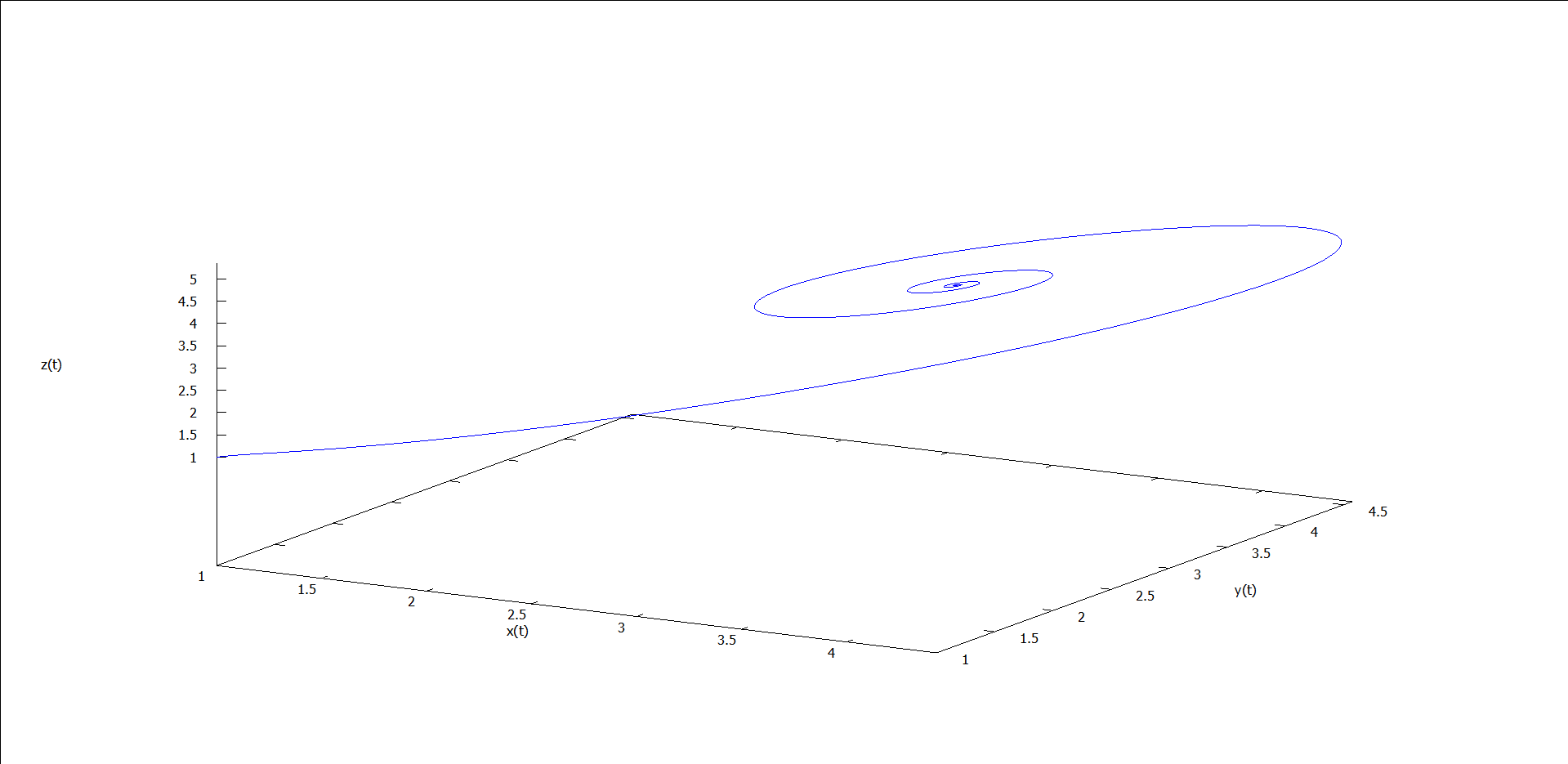图3 ρ = 5 时的运动轨线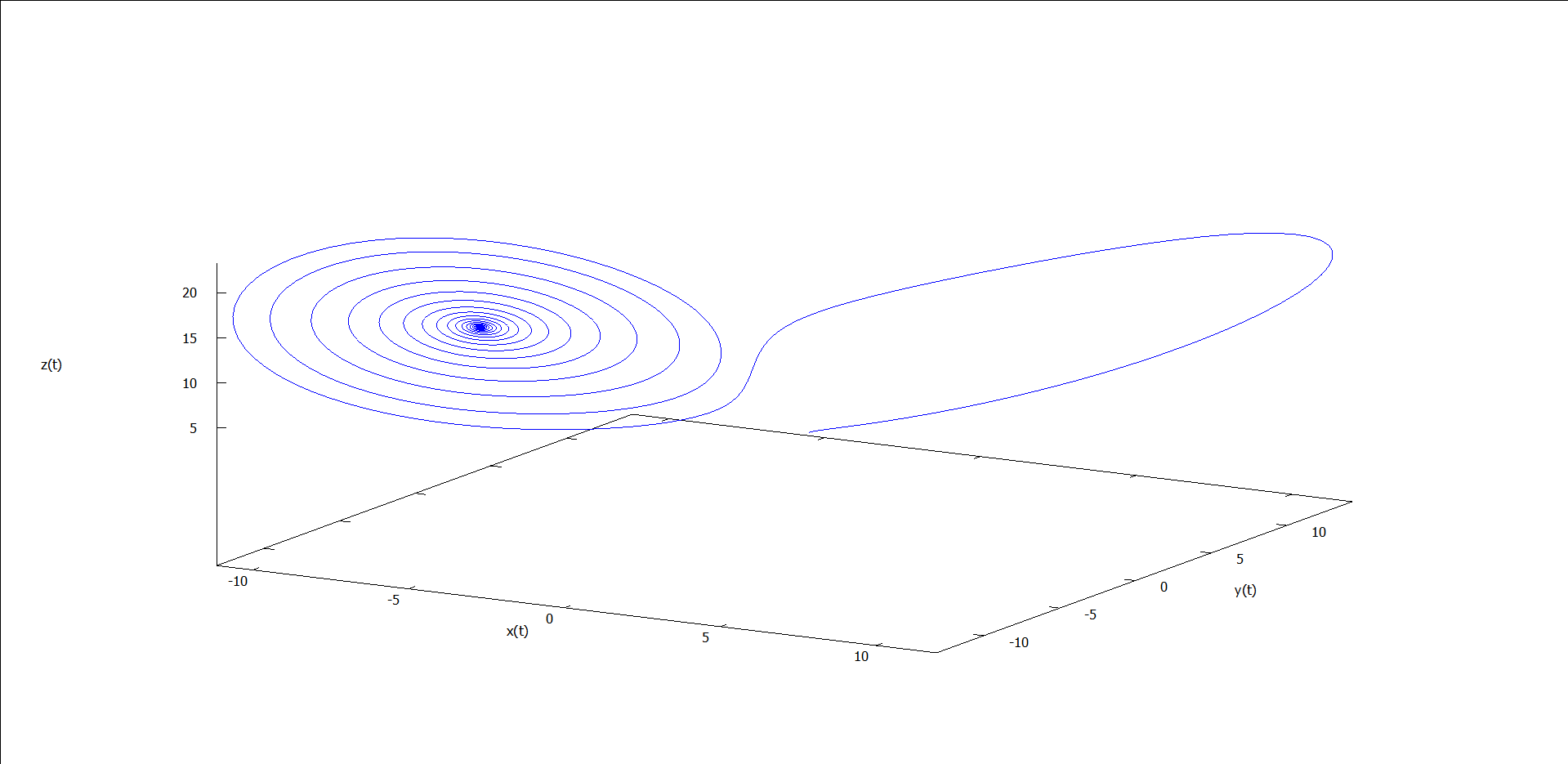图4 ρ = 15 时的运动轨线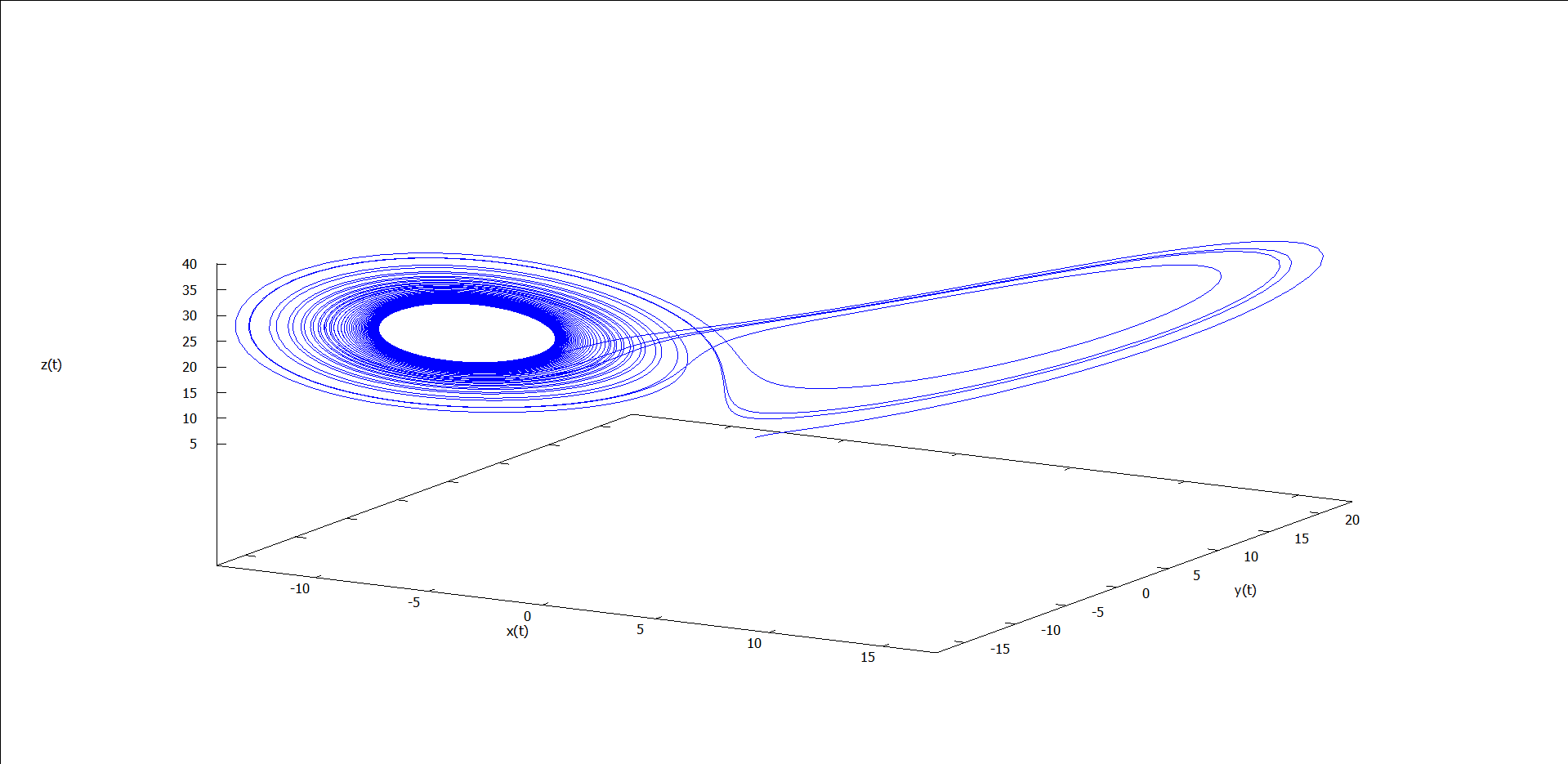图5 ρ = 24 时的运动轨线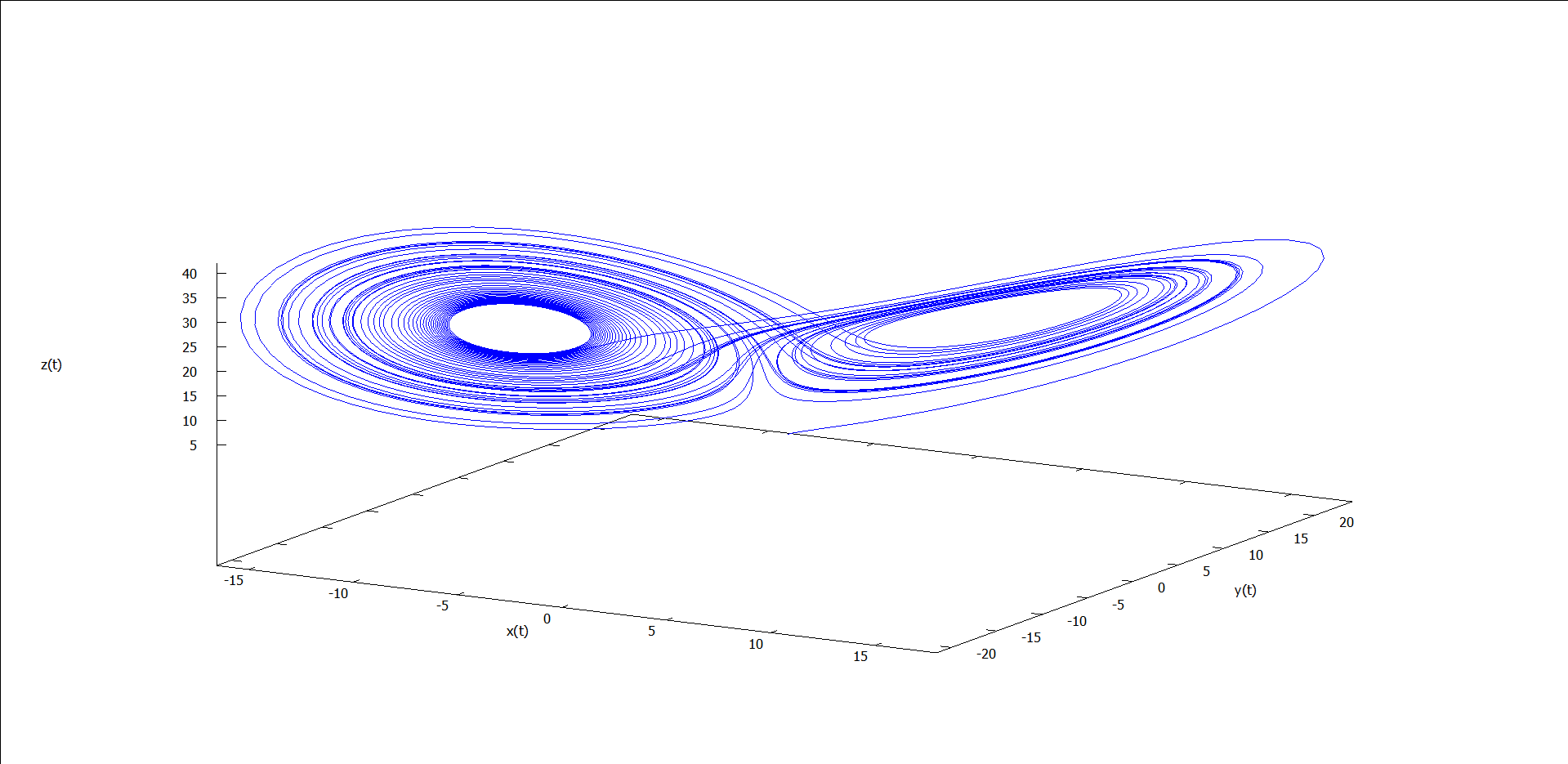图6 ρ = 25 时的运动轨线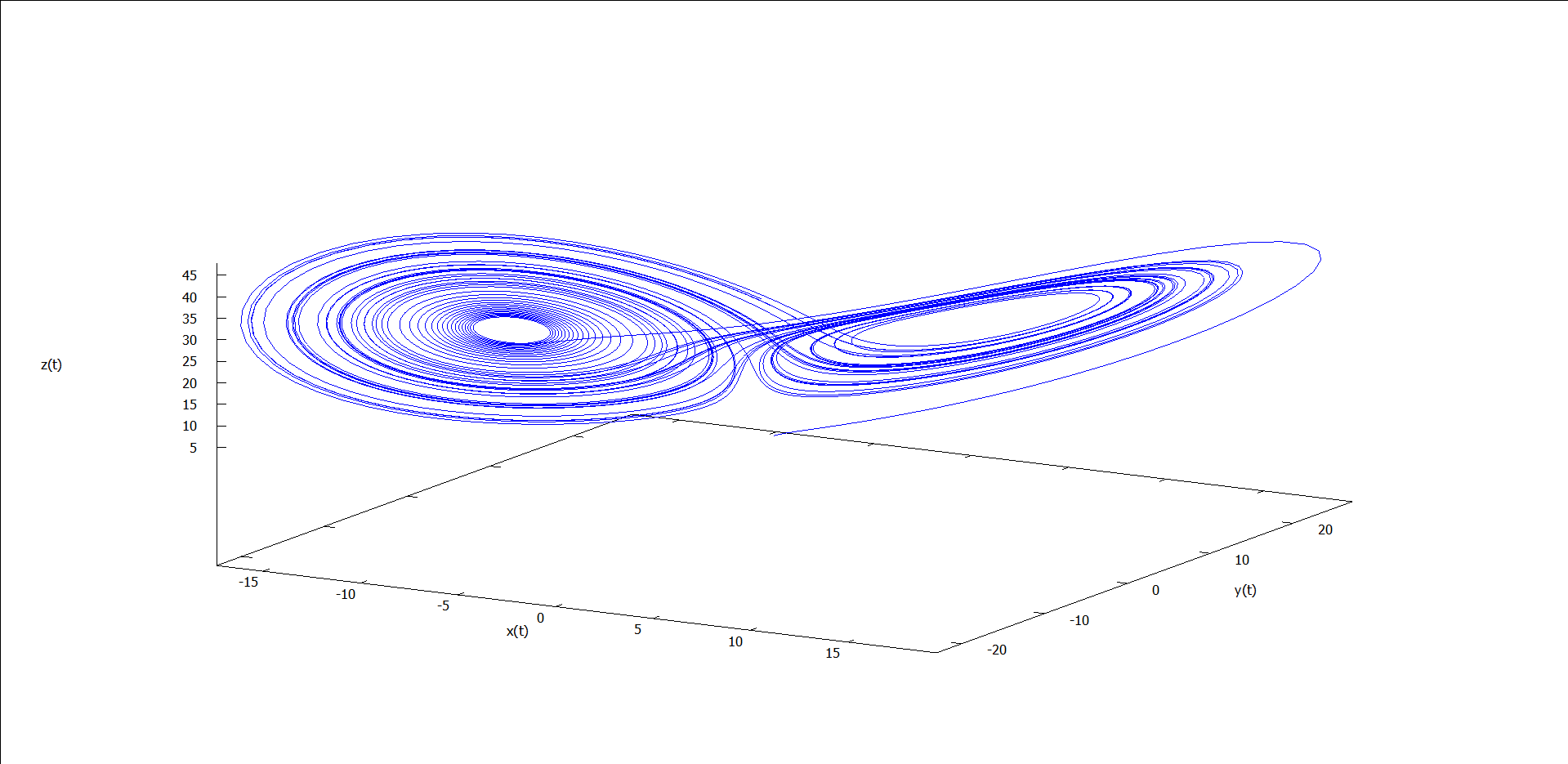图7 ρ = 28 时的运动轨线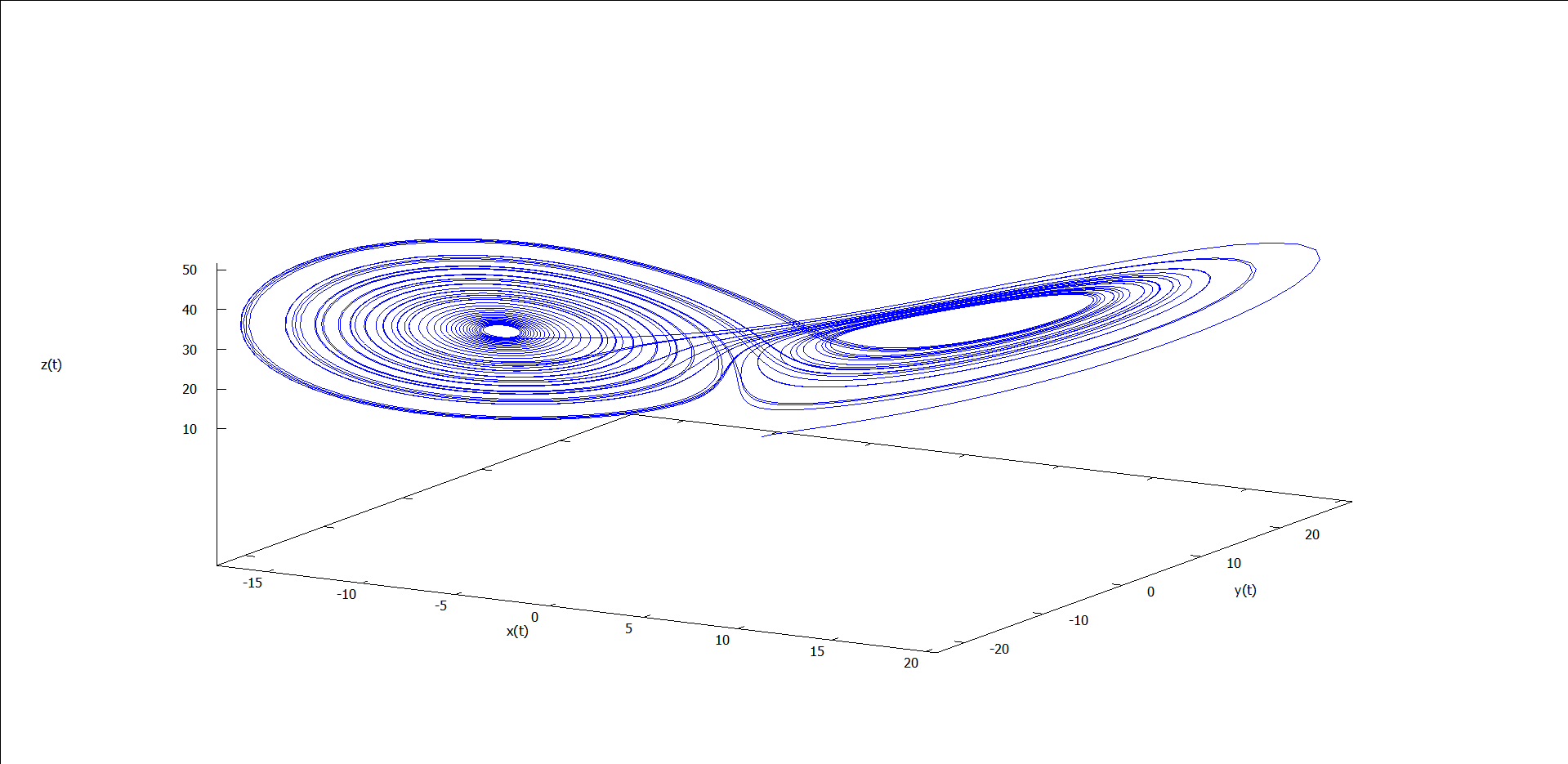图8 ρ = 30 时的运动轨线 能看出什么吗？似乎能看出点，但又不完全懂——和我一样。我们不关心这么多了，因为这些涉及复杂的微分方程求解的问题，我们只关心图7（ρ = 28）。 图7所针对的$\sigma$= 10，$\beta$= 8/3,$\rho\$ = 28 的条件正是洛伦茨论文中所探讨的。为了能进一步看清楚洛伦茨吸引子长什么样子，我们从维基百科得到一个高清版本。如图9所示，其中轨线最初从红色开始运动，并逐渐渐变为蓝色。

## 拉伸和折叠

• 拉伸：把距离局部扩张，邻近的点被扯开。
• 折叠：把拉伸后的圆绕自身折叠多次，这是用原来的圆容纳拉伸后的圆的唯一办法。这样，尽管相互靠近的点分开了，有些离得很远的点却移近了。